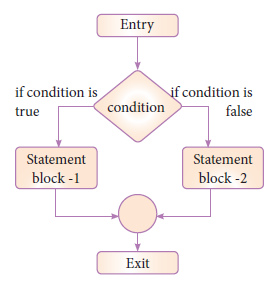#### if...else Statement in Pythpn

The if .. else statement provides control to check the true block as well as the false block. Following is the syntax of ‘if..else’ statement.

Syntax:

```if <condition>:
statements-block 1
else:
statements-block 2```if..else statement thus provides two possibilities and the condition determines which BLOCK is to be executed.

Example : #Program to check if the accepted number odd or even

``````a = int(input("Enter any number :"))
if a%2==0:
print (a, " is an even number")
else:
print (a, " is an odd number")``````

Output 1:

Enter any number :56

56 is an even number

Output 2:

Enter any number :67

67 is an odd number

An alternate method to rewrite the above program is also available in Python. The complete if..else can also written as:

Syntax:

``variable = variable1 if condition else variable 2``

Example : #Program to check if the accepted number is odd or even (using alternate method of if...else)

``````a = int (input("Enter any number :"))
x="even" if a%2==0 else "odd"
print (a, " is ",x)``````

Output 1:

Enter any number :3

3 is odd

Output 2:

Enter any number :22

22 is even